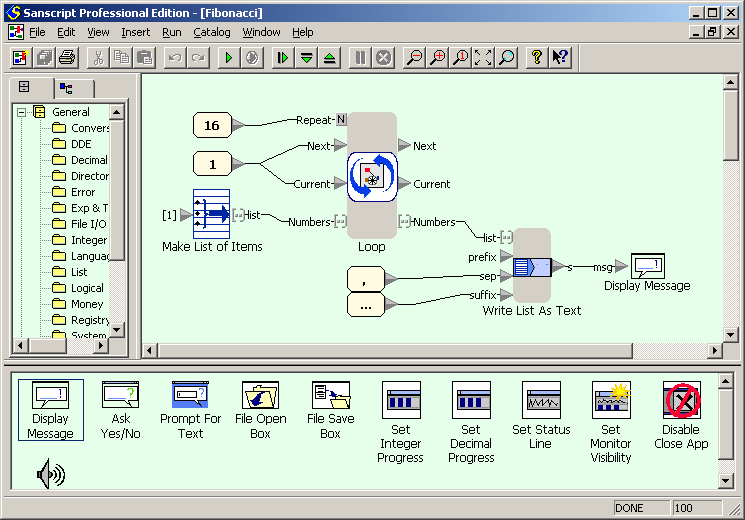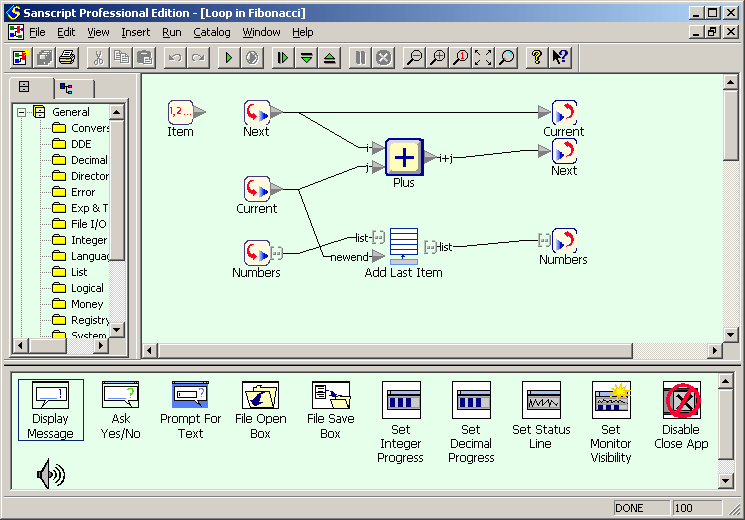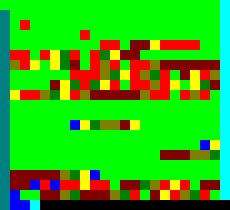# 10 unnatural ways to calculate Fibonacci numbers

Posted by Mariia Mykhailova on May 30, 2010

The task of calculating first dozen of Fibonacci numbers has lost its practical value ages ago. Nowadays it's used mostly for illustrating some basic programming techniques, recursion and iteration among them. In this writeup I will use it to show several programming languages, in which it acquires uncommon and sometimes definitely unhealthy look.

So, here goes my rating of ten most unnatural ways to calculate Fibonacci numbers of the ones I used for Progopedia project. For a more accurate definition, let's demand that the program outputs first 16 numbers, formatted as
1, 1, 2, 3, 5, 8, 13, 21, 34, 55, 89, 144, 233, 377, 610, 987, ...

#### 10. Sanscript

A visual programming language, in which all language elements are represented as elementary blocks. The "code" itself, called flowgram, is built from these blocks by connecting them with links. What placed this language in the rating is:

• the size of flowgrams: two dozens of blocks is a maximum that can be used within one flowgram without scrolling it or getting entangled in connections between blocks;
• the complexity of basic language constructs: each loop or conditional statement requires one or more separate flowgrams of description, and their numbers multiply endlessly;
• the fact that the program is not written but drawn, and the keyboard is used only for entering constant values, renaming elementary blocks and adding comments;
• and the way the flowgrams overall look like large bowls of spaghetti - talk about spaghetti code!Main flowgramLoop body

#### 9. gnuplot

The specifics of this language (up to version 4.4.0) are the absence of loops as such. This is excusable - after all, gnuplot is not a general-purpose language but a graph plotting software. A loop can be simulated by creating a separate file with loop body, which then should be "read" to start the loop and "reread" for each iteration.

run.gp (main file)

``````#!/usr/bin/env gnuplot
i = 1
a = 1
b = 1
res = ''
print res, '...'
``````

fibonacci.gp (loop body)

``````res = res . a . ', '
c = a
a = b
b = b+c
i = i+1
``````

Lazy evaluation along with infinite lists is one of the most widely known Haskell features. In this case they allow to define (but not to calculate yet) Fibonacci numbers in one line of code. The rest of code requests the required numbers and prints them in required format. Haskell is nowhere near an esoteric or unpopular language, but still this approach is far from evident or natural for someone who was raised and educated within procedural paradigm.

``````module Main where
import Text.Printf
fibs :: [Int]
fibs = 0 : 1 : zipWith (+) fibs (tail fibs)
line n = printf "%d, " \$ fibs !! n
main = do
sequence_ \$ map line [1..16]
putStrLn "..."``````

#### 7. SQL

SQL itself is not a programming language, and in most implementations it's accompanied with procedural extension which makes the task trivial. Let's try to solve the task without using procedural extensions, in "pure" SQL. Unfortunately, standard definition of "pure" SQL has nothing that can be used as a loop. Thus the solutions turn out to depend on concrete implementation and its features.

#### 7.1. Oracle SQL (since version 9i)

The innermost query generates numbers indices (from 1 to 16) using level pseudo-column and connect by construct. Next query calculates Fibonacci numbers themselves from their indices using Binet's formula. Two outermost queries order the numbers by their indices (ascending) and concatenate them in one string of required format.

``````SELECT REPLACE(MAX(SYS_CONNECT_BY_PATH(fib||', ', '/')),'/','')||'...'
FROM ( SELECT n, fib, ROW_NUMBER()
OVER (ORDER BY n) r
FROM (select n, round((power((1+sqrt(5))*0.5, n)
- power((1-sqrt(5))*0.5, n))/sqrt(5)) fib
from (select level n
from dual
connect by level <= 16) t1) t2
)
CONNECT BY PRIOR r = r-1;
``````

#### 7.2. Oracle SQL (since version 10g)

A convenient but seldom used and thus little-known operator model allows implementing a loop inside of an SQL query.

``````select max(s) || ', ...'
from
(select s
from dual
model
return all rows
dimension by ( 0 d )
measures ( cast(' ' as varchar2(200)) s, 0 f)
rules iterate (16)
(  f[iteration_number] = decode(iteration_number,
0, 1, 1, 1, f[iteration_number-1] + f[iteration_number-2]),
s[iteration_number] = decode(iteration_number,
0, to_char(f[iteration_number]),
s[iteration_number-1] || ', ' || to_char(f[iteration_number]))
)
);``````

#### 7.3. MySQL

The means of calculating query results in a loop is implemented more compactly than in Oracle.

``````select concat(group_concat(f separator ', '), ', ...')
from (select @f := @i + @j as f, @i := @j, @j := @f
from information_schema.tables, (select @i := 1, @j := 0) sel1
limit 16) t``````

#### 7.4. Microsoft SQL Server (since version 2005)

Here comes one more rather compact loop implementation, this time using a recursive query.

``````with fibonacci(a, b) as
(
select 1, 1
union all
select b, a+b from fibonacci where b < 1000
)
SELECT cast(a as varchar)+', ' AS [text()]
FROM fibonacci
FOR XML PATH ('')``````

#### 6. FP

FP is a prototype of all existing programming languages which use function-level paradigm (not to be confused with functional). Created in 1977, it is more of a math model than of a real language: it had no official standard (except for one article, in which it was actually suggested), not to mention working interpreters! However, nowadays there exists a number of FP interpreters, usually written as a student work. One of them is Furry Paws, and its cozy name efficiently hides the inconvenience of using it.

Function-level programming states that the program is built from elementary functions, combined with function-to-function operations. Thus, ~1 is an elementary function which always returns 1; id returns the value it accepted, and [] is a functional form which combines its arguments in a sequence.

``````one = eq.[id, ~1]
dec = sub.[id, ~1]
seq = one -> [~1] ; cat.[seq.dec, [id]]
fibonacci = lt.[id, ~3] -> ~1 ; add.[fibonacci.sub.[id, ~1], fibonacci.sub.[id, ~2]]

main = show.(return @fibonacci.(seq.~16))
``````

#### 5. J

J is one more function-level language, a worthy successor of FP. One of features of this language is that nearly every phrase in it can be written using several ways, from "almost traditional" to absolutely unnatural (and that's what is needed in this article). Thus, for example, using Binet's formula can be written quite nicely:

``````load 'printf'
g =: 0.5 * (1 + 5 ^ 0.5)
fib =: (0.2 ^ 0.5) * (g &^ - (1-g) &^)
fstr=: '...' ,~ ,~^:4 '%d, '
fstr printf fib 1+i.16
``````

Or you can replace all math operators with their J-specific equivalents:

``````load 'printf'
g =: -: >: %:5
fib =: (%:5) %~ g&^ - (1-g)&^
fstr =: '...' ,~ ,~^:4 '%d, '
fstr printf fib"0 >:i.16
``````

And hardly anybody will think it evident that %: is square-rooting, >: is increment, -: is halving, and %~ is division, with dividend and divisor swapped.

Recursion-based calculation:

``````load 'printf'
fibr=: 1:`(-&2 + &fibr -&1) @.(2&<)"0
fstr=: '...' ,~ ,~^:4 '%d, '
fstr printf fibr 1+i.16
``````

#### 4. Hanoi Love

A little-known esoteric language, interesting by its minimalism and usage of stack-based memory model. Unlike next language, the trickiest part of programming is not executing math operations but extracting the contents of the stack elements needed on each step. Printing results, however, is quite an unpleasant task, so only first six one-digit numbers are calculated and printed.

``````;;;;;;;;;;;;;;;;;;;;;;;;;;;;;;;;.'...;;;;;;;;;;;;.'...,..''''''..`.'.
..;.'.,.'...:...,.'..;;;;;;;;;;;;;;;;;;;;;;;;;;;;;;;;;;;;;;;;;;;;;;;;
"'.,.'...,"'.'...,"''.,...'.,..'..,...'...;.'.,.,!...,,,...;;"'"'"'
``````

Language description and comments for the given code can be found at the page of Hanoi Love.

#### 3. Brainfuck

The classics of esoteric programming - Brainfuck, a language in which even assignment or addition is not an elementary operation, printing three-digit number deserves a separate ode, and using it for solving any practical task is masochist's dream :-)

In original interpreter (Muller's Brainfuck) memory cells are of type byte, so Fibonacci numbers starting from 14th would cause a memory overflow. Implementing long arithmetic in Brainfuck is not a symptom but rather a diagnosis, so I assumed that a memory cell can store numbers up to at least 1000 (a lot of implementations use data types capacious enough).

``````++++++++++++++++++++++++++++++++++++++++++++>++++++++++++++++++++++++
++++++++>++++++++++++++++>>+<<[>>>>++++++++++<<[->+>-[>+>>]>[+[-<+>]>
+>>]<<<<<<]>[<+>-]>[-]>>>++++++++++<[->-[>+>>]>[+[-<+>]>+>>]<<<<<]>[-
]>>[++++++++++++++++++++++++++++++++++++++++++++++++.[-]]<[++++++++++
++++++++++++++++++++++++++++++++++++++.[-]]<<<+++++++++++++++++++++++
+++++++++++++++++++++++++.[-]<<<<<<<.>.>>[>>+<<-]>[>+<<+>-]>[<+>-]<<<
-]<<++...
``````

Language description and comments for the given code can be found at the page Brainfuck.

#### 2. Brainloller

This is the simplest of graphical dialects of Brainfuck, invented by Lode Vandevenne. The programs are read from PNG images, the commands are written as pixels of different colors, and the set of 8 Brainfuck commands is extended with two more which control the direction of pointer to the current pixel. This language inherits all the delights of coding in Brainfuck and has some of its own, for example, absolute unreadability of the "code" and impossibility of coding without supplements like code-image converter.

Unfortunately, the original interpreter of the language sank into oblivion, so to generate this "program" I had to write a tool of my own. The image is given in tenfold magnification.#### 1. Unary

Can it be worse? - the reader will think while scrolling the text with trembling hand. Yes, it can. In all mentioned languages the program could be at least viewed and envisioned, and that's a lot.

Meet the winner of the rating - Unary. This dialect of Brainfuck (invented by Lode Vandevenne again) requires that Brainfuck commands are converted to binary codes and concatenated into a single binary number. Unary notation of this number is the code in Unary. This way the code which generates Fibonacci numbers is a string of

``````167967665105731198557055496639385943332278803897935697536099438828197
665241403160165880863622431582784595268769268183940269756210147305655
704025762911607244068691728105306566342622386432823429136972542304655
647901781271798433263001837026612851345264031562174039657802748245705
398528237993320520942720239597540583536934220029626573406470088757427
393143000966310611249037587993216365993804186165097620168960460854977
571944373603975793034586829061577464233522714007498991416860375267535
193648636795096472789203729505034887001634966681420589637468649908257
407260923590831776308356684326657774592110098643361324426156431864437
942781495979555960608253552679248495326880775320385281559763269974848
026839024530519989287202261977272377723622502479809174132505837648641
033569945906182518892142219706483917757108086522763280388915772444727
238483811923456440363260610571471034139736312976255142288379411989404
9017738035 (approximately 1.68*10^906) zeros.
``````

I'm pretty sure that the languages given here are not the worst ones a programmer can face (well, at least not all of the worst ones), but those will come later.